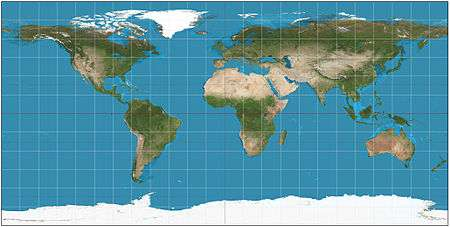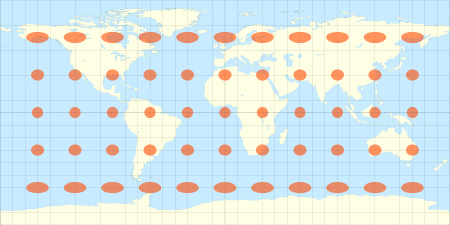# Equirectangular projectionEquirectangular projection of the world; the standard parallel is the equator (plate carrée projection).The equirectangular projection with Tissot's indicatrix of deformation

The equirectangular projection (also called the equidistant cylindrical projection, geographic projection, or la carte parallélogrammatique projection, and which includes the special case of the plate carrée projection or geographic projection) is a simple map projection attributed to Marinus of Tyre, who Ptolemy claims invented the projection about AD 100. The projection maps meridians to vertical straight lines of constant spacing (for meridional intervals of constant spacing), and circles of latitude to horizontal straight lines of constant spacing (for constant intervals of parallels). The projection is neither equal area nor conformal. Because of the distortions introduced by this projection, it has little use in navigation or cadastral mapping and finds its main use in thematic mapping. In particular, the plate carrée has become a standard for global raster datasets, such as Celestia and NASA World Wind, because of the particularly simple relationship between the position of an image pixel on the map and its corresponding geographic location on Earth.

## Definition

The forward projection transforms spherical coordinates into planar coordinates. The reverse projection transforms from the plane back onto the sphere. The formulae presume a spherical model and use these definitions:

λ is the longitude of the location to project;
φ is the latitude of the location to project;
φ1 are the standard parallels (north and south of the equator) where the scale of the projection is true;
λ0 is the central meridian of the map;
x is the horizontal coordinate of the projected location on the map;
y is the vertical coordinate of the projected location on the map.

### ForwardThe plate carrée (French, for flat square), is the special case where φ1 is zero. This projection maps x to be the value of the longitude and y to be the value of the latitude, and therefore is sometimes called the latitude/longitude or lat/lon(g) projection or is said (erroneously) to be “unprojected”.

While a projection with equally spaced parallels is possible for an ellipsoidal model, it would no longer be equidistant because the distance between parallels on an ellipsoid is not constant. More complex formulae can be used to create an equidistant map whose parallels reflect the true spacing.

### Reverse